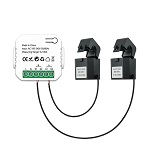# # TuYa PJ-1203A

ModelPJ-1203A
VendorTuYa
DescriptionBidirectional energy meter with 80A current clamp
Exposesac_frequency, voltage, power_a, power_b, power_ab, current_a, current_b, power_factor_a, power_factor_b, energy_flow_a, energy_flow_b, energy_a, energy_b, energy_produced_a, energy_produced_b, update_frequency, linkquality
Picture## # Options

How to use device type specific configuration

• `voltage_precision`: Number of digits after decimal point for voltage, takes into effect on next report of device. The value must be a number with a minimum value of `0` and with a with a maximum value of `3`

• `voltage_calibration`: Calibrates the voltage value (percentual offset), takes into effect on next report of device. The value must be a number.

## # Exposes

### # AC frequency (numeric)

Measured electrical AC frequency. Value can be found in the published state on the `ac_frequency` property. It's not possible to read (`/get`) or write (`/set`) this value. The unit of this value is `Hz`.

### # Voltage (numeric)

Measured electrical potential value. Value can be found in the published state on the `voltage` property. It's not possible to read (`/get`) or write (`/set`) this value. The unit of this value is `V`.

### # Power a (numeric)

Instantaneous measured power (phase A). Value can be found in the published state on the `power_a` property. It's not possible to read (`/get`) or write (`/set`) this value. The unit of this value is `W`.

### # Power b (numeric)

Instantaneous measured power (phase B). Value can be found in the published state on the `power_b` property. It's not possible to read (`/get`) or write (`/set`) this value. The unit of this value is `W`.

### # Power ab (numeric)

Instantaneous measured power (phase AB). Value can be found in the published state on the `power_ab` property. It's not possible to read (`/get`) or write (`/set`) this value. The unit of this value is `W`.

### # Current a (numeric)

Instantaneous measured electrical current (phase A). Value can be found in the published state on the `current_a` property. It's not possible to read (`/get`) or write (`/set`) this value. The unit of this value is `A`.

### # Current b (numeric)

Instantaneous measured electrical current (phase B). Value can be found in the published state on the `current_b` property. It's not possible to read (`/get`) or write (`/set`) this value. The unit of this value is `A`.

### # Power factor a (numeric)

Instantaneous measured power factor (phase A). Value can be found in the published state on the `power_factor_a` property. It's not possible to read (`/get`) or write (`/set`) this value. The unit of this value is `%`.

### # Power factor b (numeric)

Instantaneous measured power factor (phase B). Value can be found in the published state on the `power_factor_b` property. It's not possible to read (`/get`) or write (`/set`) this value. The unit of this value is `%`.

### # Energy flow a (enum)

Direction of energy (phase A). Value can be found in the published state on the `energy_flow_a` property. It's not possible to read (`/get`) or write (`/set`) this value. The possible values are: `consuming`, `producing`.

### # Energy flow b (enum)

Direction of energy (phase B). Value can be found in the published state on the `energy_flow_b` property. It's not possible to read (`/get`) or write (`/set`) this value. The possible values are: `consuming`, `producing`.

### # Energy a (numeric)

Sum of consumed energy (phase A). Value can be found in the published state on the `energy_a` property. It's not possible to read (`/get`) or write (`/set`) this value. The unit of this value is `kWh`.

### # Energy b (numeric)

Sum of consumed energy (phase B). Value can be found in the published state on the `energy_b` property. It's not possible to read (`/get`) or write (`/set`) this value. The unit of this value is `kWh`.

### # Energy produced a (numeric)

Sum of produced energy (phase A). Value can be found in the published state on the `energy_produced_a` property. It's not possible to read (`/get`) or write (`/set`) this value. The unit of this value is `kWh`.

### # Energy produced b (numeric)

Sum of produced energy (phase B). Value can be found in the published state on the `energy_produced_b` property. It's not possible to read (`/get`) or write (`/set`) this value. The unit of this value is `kWh`.

### # Update frequency (numeric)

Update frequency. Value can be found in the published state on the `update_frequency` property. It's not possible to read (`/get`) or write (`/set`) this value. The unit of this value is `s`.

Link quality (signal strength). Value can be found in the published state on the `linkquality` property. It's not possible to read (`/get`) or write (`/set`) this value. The minimal value is `0` and the maximum value is `255`. The unit of this value is `lqi`.